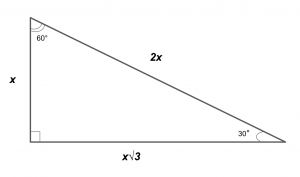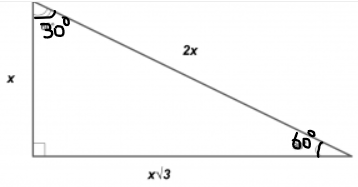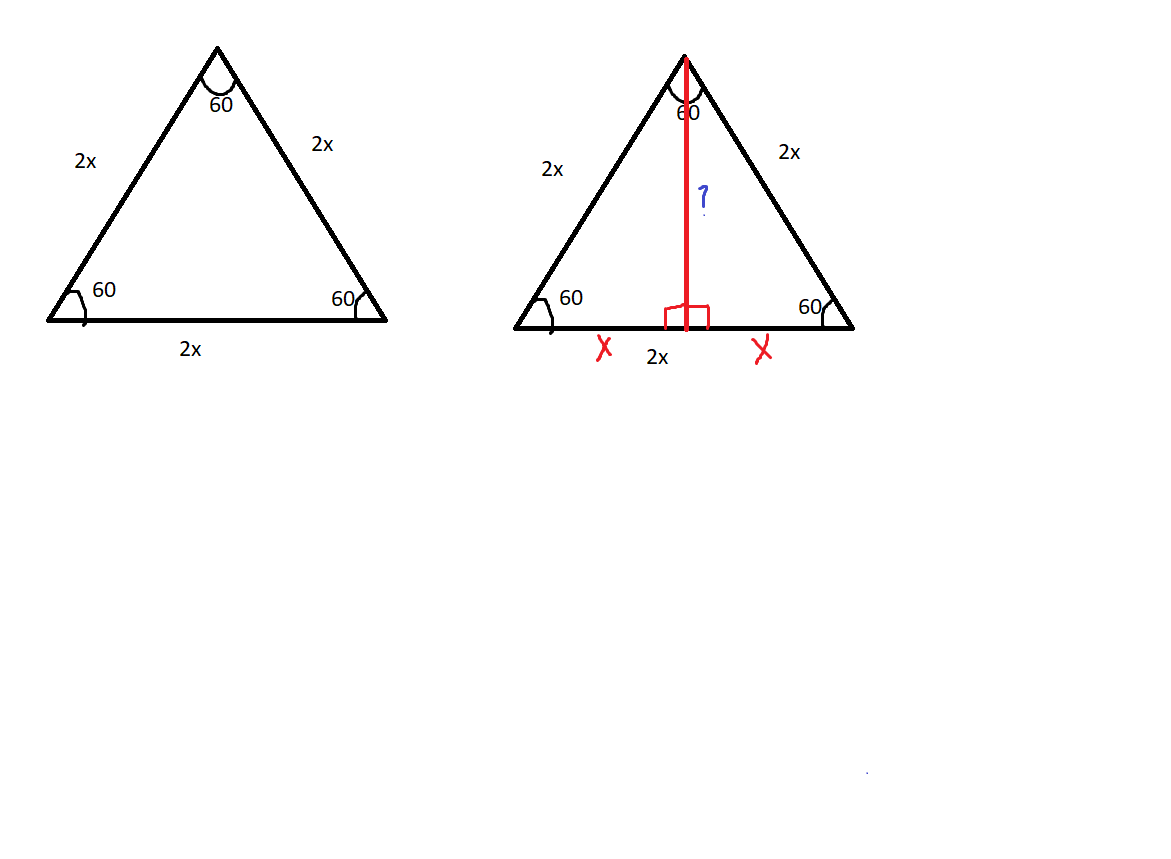# Why can't the height of the new "mail" shape be 3? Since the middle line is already three?

• And I kinda need more explanation on how the height is 3/2 square root of 3.....

• @victorioussheep To answer your second question, we can figure out the height using the special properties of a 30-60-90 triangle! When you have a triangle with angles 30, 60, and 90 degrees, and you know the length of one of its sides, then you can figure out the lengths of all the other sides (this is why this type of triangle is really useful):So in this case, we have a 30-60-90 triangle, and the hypotenuse is given to be 3. So then we know that the other sides have length (3/2) and (3/2)*sqrt(3).

You definitely don't have to memorize this-- if you understand how the proof for how the lengths are derived (hint: drop an altitude from an equilateral triangle) and use the fact a few times, it becomes pretty intuitive.

For your first question, the height of the new "mail" shape isn't 3 because the segment with line 3 isn't perpendicular to the other diagonal. Remember that the "height" of something always has to form a 90 degree angle with the base! This is basically the same ideas as why, if you have some triangle ABC, drawing any line from A to hit BC isn't necessarily the height of ABC. The height is only the line from A that is perpendicular to BC.

I hope this helped!• @audrey so......let me get this straighter...the x that you have used in that picture can be ANY number, right? so then does that mean that if x is 2, then the longest side of that triangle is 4? And the bottom side is 2sqrt(3)?

• @audrey Then would it possible if your picture looks somewhat like this:would that be the same as the method?

• @victorioussheep Yes, x just represents any number! So you're correct-- if x=2, then the hypotenuse has length 2 * 2 = 4, and the other leg has length 2*sqrt(3).For your second question, not exactly... the side length with length xsqrt(3) always must be adjacent to the 30 degree angle! That picture isn't exactly drawn to scale, but with any 30-60-90 triangle, one of the sides (the one with length xsqrt(3)) is always longer than the other. This diagram might help:Basically, by dropping an altitude from an equilateral triangle, you can see that the shorter side (the one between 60 degrees and the right angle) always has length x. And then you can use the Pythagorean theorem to solve for the blue ?.

Does that make more sense?

• @audrey hmm......A little bit not understandable but also somehow makes sense...

• @victorioussheep lolwhich part specifically doesn't make sense? I can try to explain it clearer.

• @audrey Oh...what I meant is that I understand it in a weird way....but it makes sense and I get that explanation• @victorioussheep Oh okay, sounds good!